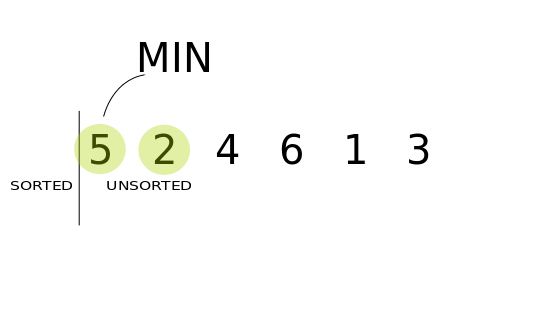## Selection Sort in JavaScript

### Introduction

Selection Sort is one of the simpler and more intuitive sorting algorithms. It is an in-place, unstable, comparison algorithm.

This means that it transforms the input collection using no auxiliary data structures and that the input is overridden by the output (in-place algorithm).

Additionally, during its execution, it only reads list elements through a single abstract comparison operation, usually a "less than or equal to" operator (comparison algorithm).

Finally, the order of duplicate elements isn't preserved after sorting (unstable algorithm).

It is usually taught early on in most Computer Science courses, given that it is very easy to understand and translate into code. Despite its quadratic time complexity, it has performance advantages over some more complex sorting algorithms, such as Quicksort or Merge Sort, in some specific cases.

In this article, we will explain the idea behind Selection Sort and implement it in JavaScript.

After that, we'll analyze its time complexity and compare it to other sorting algorithms. Finally, we will discuss when it can be used, as well as when it should be avoided.

### Selection Sort

This algorithm divides the input array into two sublists - a sorted and unsorted sublist. The sorted list is located at the start of the collection, and all elements to the right of the final sorted element are considered unsorted.

Initially, the sorted list is empty, while the rest of the collection is unsorted. Selection Sort goes through the unsorted sublist and finds the smallest or largest element.

The element is then swapped with the leftmost element of the unsorted sublist. Then, the sorted sublist is expanded to include that element.

This is repeated, finding the fitting element, swapping it with the leftmost element of the unsorted list, and expanding the sorted list to encompass it.

After each iteration, one less element needs to be checked, until the entire array or list is sorted. In other words, after the k-th iteration, the first k elements of the array or list are guaranteed to be sorted.

Let's look at this visual representation of how Selection Sort works on the array of:

 5, 2, 4, 6, 1, 3### Selection Sort Implementation

Now that we've gotten over the idea behind Selection Sort and its visual representation, we can move on to the algorithm implementation in JavaScript:

function selectionSort(inputArr) {
let n = inputArr.length;

for(let i = 0; i < n; i++) {
// Finding the smallest number in the subarray
let min = i;
for(let j = i+1; j < n; j++){
if(inputArr[j] < inputArr[min]) {
min=j;
}
}
if (min != i) {
// Swapping the elements
let tmp = inputArr[i];
inputArr[i] = inputArr[min];
inputArr[min] = tmp;
}
}
return inputArr;
}


We're iterating through the entire input array, and for each element of the array - we find the smallest number in the unsorted subarray. If the smallest number isn't the first one in the unsorted subarray (isn't already in its place), we swap it with the first element of the unsorted subarray.

Without checking if the smallest element is already the first element of the unsorted subarray, we'd be performing unnecessary swaps.

Now, let's add the input array and invoke our function:

let inputArr = [5, 2, 4, 6, 1, 3];
selectionSort(inputArr);
console.log(inputArr);


The output of this code will be:

(6) [1, 2, 3, 4, 5, 6]


### Time Complexity

The time complexity of Selection Sort is not difficult to analyze.

In the first iteration, throughout the array of n elements, we make n-1 comparisons and potentially one swap. In the second iteration, we will make n-2 comparisons, and so on.

Therefore, the total number of comparisons will be (n-2) + (n-1) + ...+ 1, which adds up to n(n-1)/2 = (n2-n)/2. This leads us to a running time of O(n2).

O(n2) is a pretty bad time complexity for a sorting algorithm. When sorting a collection, you'd use faster sorting algorithms like Quicksort or Merge Sort with a time complexity of O(nlogn).

The best-case performance of Selection Sort is also O(n2), so checking whether the array or list is sorted or not is also really inefficient.

On the other hand, when compared to other quadratic time complexity algorithms like Bubble Sort, Selection Sort holds itself quite well, outperforming Bubble Sort and its variants, as well as Gnome Sort.

Insertion sort, however, can be faster than Selection Sort in case the collection is almost sorted. And Insertion Sort is pretty much unbeaten with short collections.

### Selection Sort Variants

Even though the best, worst and average-case performances of this algorithm are O(n2), making it pretty inefficient, Selection Sort is very important for the overall development of sorting algorithms. It was, in fact, a base for some very widely used and efficient sorting algorithms!

Perhaps the most famous variant is Heap Sort, which internally uses a heap data structure for storing elements. Using heaps can speed up the finding and removal of elements to an O(logn) time.

This is a very beneficial optimization that reduces the total running time from O(n^2) to O(nlogn), which is considered good for sorting algorithms.

Another variant is the BidirectionaL Selection Sort, similar to how Bubble Sort has a bidirectional counterpart. It's sometimes known as Cocktail Sort (again, similar to Bubble's Cocktail Shaker Sort), and does the same job in ~25% fewer comparisons.

### Conclusion

Selection Sort is a fundamental and simple, thus inefficient sorting algorithm. It served as a basis for some of the widely-used and adopted algorithms and isn't used very often itself in the development industry.

However, if the input collection is small, Selection Sort is a better option than Quicksort or Merge Sort. But then again, Insertion Sort has shown to be more effective than Selection Sort in these cases.

In this article, we've gone over the idea behind Selection Sort, implemented it in JavaScript, explored its time complexity and briefly mentioned some of its variants.